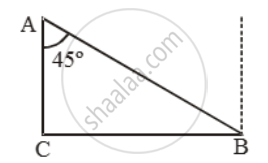# The Banks of a River Are Parallel. a Swimmer Starts from a Point on One of the Banks and Swims in a Straight Line Inclined to the Bank at 45º and Reaches the Opposite Bank at a Point 20 M from the - Mathematics

Sum

The banks of a river are parallel. A swimmer starts from a point on one of the banks and swims in a straight line inclined to the bank at 45º and reaches the opposite bank at a point 20 m from the point opposite to the starting point. Find the breadth of the river

#### Solution

Let A be the starting point and B, the end point of the swimmer. Then AB = 20 m and ∠BAC = 45º.\text{Now, }\frac{BC}{AB}=\text{sin}45^\text{o}=\frac{1}{\sqrt{2}}

\Rightarrow \frac{BC}{20}=\frac{1}{\sqrt{2}}

\Rightarrow BC=\frac{20\times \sqrt{2}}{2}=14.14\m

Concept: Heights and Distances
Is there an error in this question or solution?